# sklearn.datasets.make_circles¶

sklearn.datasets.make_circles(n_samples=100, shuffle=True, noise=None, random_state=None, factor=0.8)[source]

Make a large circle containing a smaller circle in 2d.

A simple toy dataset to visualize clustering and classification algorithms.

Read more in the User Guide.

Parameters: n_samples : int, optional (default=100) The total number of points generated. If odd, the inner circle will have one point more than the outer circle. shuffle : bool, optional (default=True) Whether to shuffle the samples. noise : double or None (default=None) Standard deviation of Gaussian noise added to the data. random_state : int, RandomState instance or None (default) Determines random number generation for dataset shuffling and noise. Pass an int for reproducible output across multiple function calls. See Glossary. factor : 0 < double < 1 (default=.8) Scale factor between inner and outer circle. X : array of shape [n_samples, 2] The generated samples. y : array of shape [n_samples] The integer labels (0 or 1) for class membership of each sample.

## Examples using sklearn.datasets.make_circles¶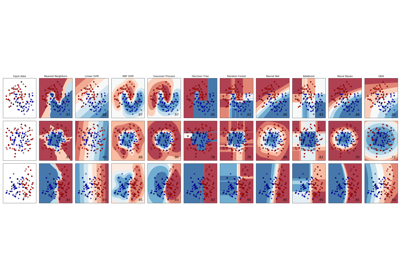Classifier comparison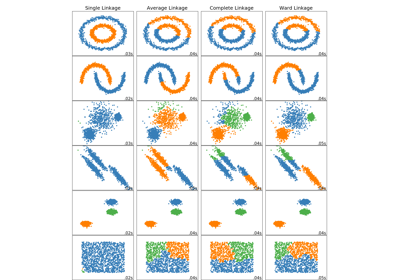Comparing different hierarchical linkage methods on toy datasets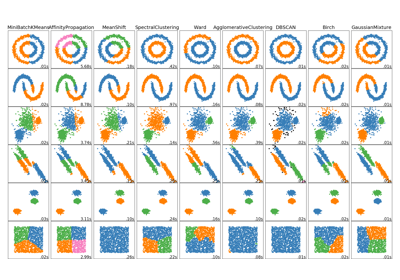Comparing different clustering algorithms on toy datasets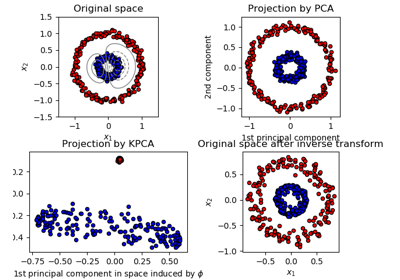Kernel PCA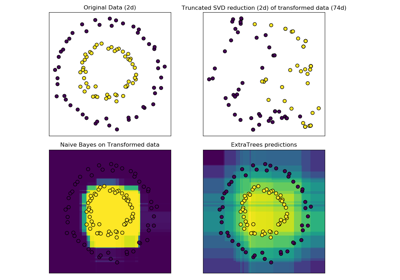Hashing feature transformation using Totally Random Trees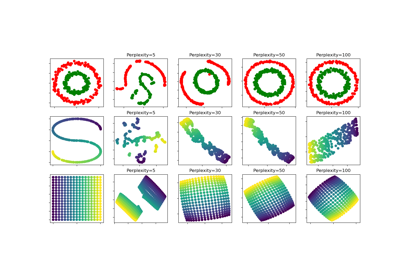t-SNE: The effect of various perplexity values on the shape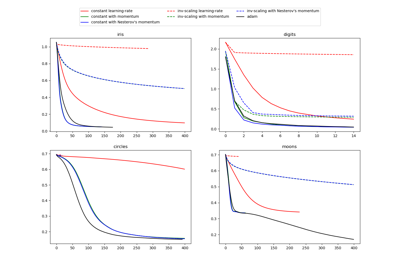Compare Stochastic learning strategies for MLPClassifier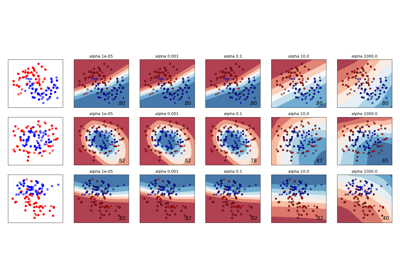Varying regularization in Multi-layer Perceptron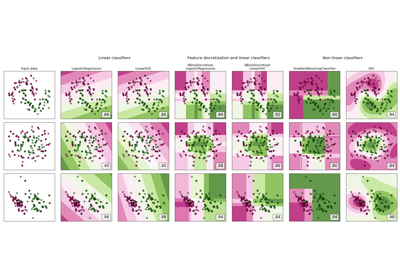Feature discretization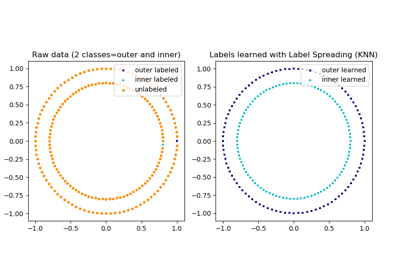Label Propagation learning a complex structure